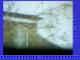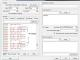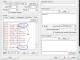Handshake on serial data
John Wood from United Kingdom  [67 posts]
9 years
 Hi, I am using the RR server and passing key button action using the default "move" variable to my Picaxe. It nearly works OK but would like an handshake between the Pic and RR . I would like Verification that the data has been received before sending the next. I use this on the Picaxe for reading the data and works, serrxd ,b6   but not really acquainted with serial data comms is it possible too communicated both ways using RR server. I keep reading on the Picaxe and it seems possible but can not grasp it. An example would be great so that I can learn by adopting or changing as I require. JohnSteven from United States  [1426 posts] 9 years
 John, If you can post your robofile we can make the needed changes. What response would you want to send from the PIC? The way you do this is to use a single variable in the Send Sequence and clear that variable immediately after the serial module ... which will ensure that the data is only sent once. Then you will have to have another variable specified in the Receive Sequence that will wait for the response from the PIC. Finally, you will have to update whatever code you are using (maybe VBScript?) that will only set the send variable again once the receive variable contains the right value. Its not very complex but does require an if statement/code in order to enable/disable sending of commands. See the attached robofile for how this is done. Note this is untested (don't have a PIC around right now) so their might be something not quite right ... but it should give you an idea on how to proceed. STeven.program.robo
John Wood from United Kingdom  [67 posts] 9 years
 Thank Steven Here is the basic I used to test the web page, RR server and response of the PIC driving the servo's, basic but will use math after I iron out the comms. '********************************************************************* 'SERVO POSITIONS '******************************************************************** symbol midH_pos  =150 symbol midV_pos  =112 symbol servo_moves =5 int: b1=midH_pos servo 3,b1 b2=midV_pos servo 2,b2 pan:                reconnect serrxd ,b6 disconnect if b6=111 then b1=195 endif if b6=110 then b1=192 endif if b6=109 then b1=189 endif if b6=108 then b1=186 endif if b6=107 then b1=183 endif if b6=106 then b1=180 endif if b6=105 then b1=177 endif if b6=104 then b1=174 endif if b6=103 then b1=171 endif if b6=102 then b1=168 endif if b6=101 then b1=165 endif if b6=100 then b1=162 endif if b6=99 then b1=159 endif if b6=98 then b1=156 endif if b6=97 then b1=153 endif if b6=48 then b1=150 endif if b6=65 then b1=147 endif if b6=66 then b1=144 endif if b6=67 then b1=141 endif if b6=68 then b1=138 endif if b6=69 then b1=135 endif if b6=70 then b1=132 endif if b6=71 then b1=129 endif if b6=72 then b1=126 endif if b6=73 then b1=123 endif if b6=74 then b1=120 endif if b6=75 then b1=117 endif if b6=76 then b1=114 endif if b6=77 then b1=111 endif if b6=78 then b1=108 endif if b6=79 then b1=105 endif '**Vertical************************* if b6=53 then b2=125 endif if b6=52 then b2=117 endif if b6=51 then b2=115 endif if b6=50 then b2=113 endif if b6=49 then b2=112 endif if b6=54 then b2=111 endif if b6=55 then b2=110 endif if b6=56 then b2=108 endif if b6=57 then b2=104 endif servo 3,b1 sertxd( "b1 = ", #b1,13 )'**Used to check Servo position servo 2,b2 sertxd( "b2 = ", #b2,13 )'**Used to check Servo position goto pan It works most of the time OK the Ascii data sent from the browser is confirmed shown on the Serial console but occasionally  the Servo position variable is not updated, usually OK if another position is chosen... All the control code for now is by the Pic so only using the Browser/Serial module of RR. Included is a capture of the browser test interface to help see what is going on.John Wood from United Kingdom  [67 posts] 9 years
 Screen shot of what I see in the Serial Module, one with error and one OK Hope it helps JohnJohn Wood from United Kingdom  [67 posts] 9 years
 Thanks again Steven, all sorted after a bit of juggling with the RR you sent. Just another point, is it possible to show how many users are viewing on the browser showing in the browser? Cheers JohnSteven from United States  [1426 posts] 9 years
 John, There is now an WEBSERVER_ACTIVE_CONNECTIONS variable (v2.47.15) that is set with the number of connections currently on the webserver ... this is not exactly how many users are connected but should be a good approximation. You can check how the image_count variable is updated to also grab that variable and display it somewhere on the page. STeven.

 This forum thread has been closed due to inactivity (more than 4 months) or number of replies (more than 50 messages). Please start a New Post and enter a new forum thread with the appropriate title.